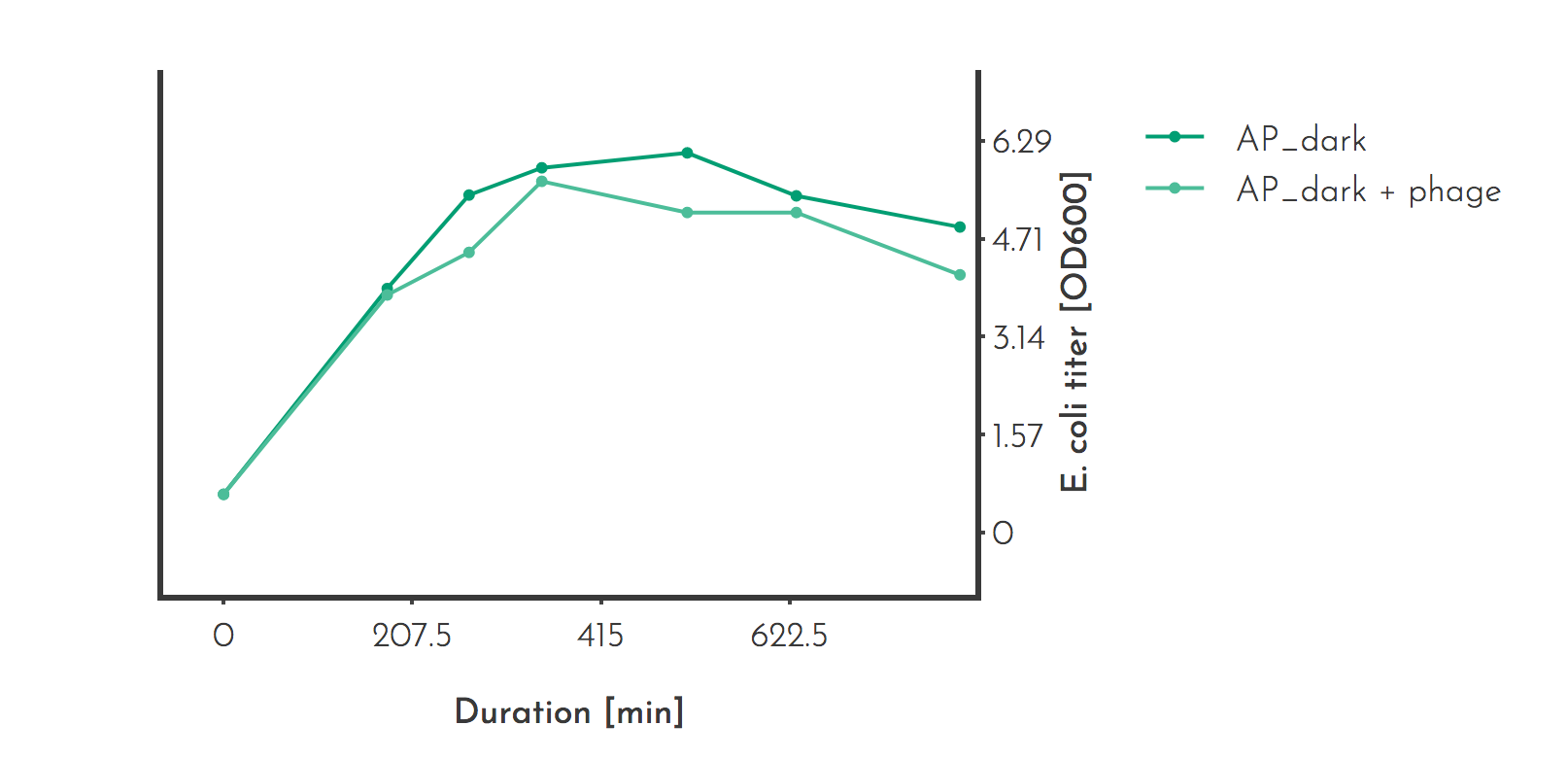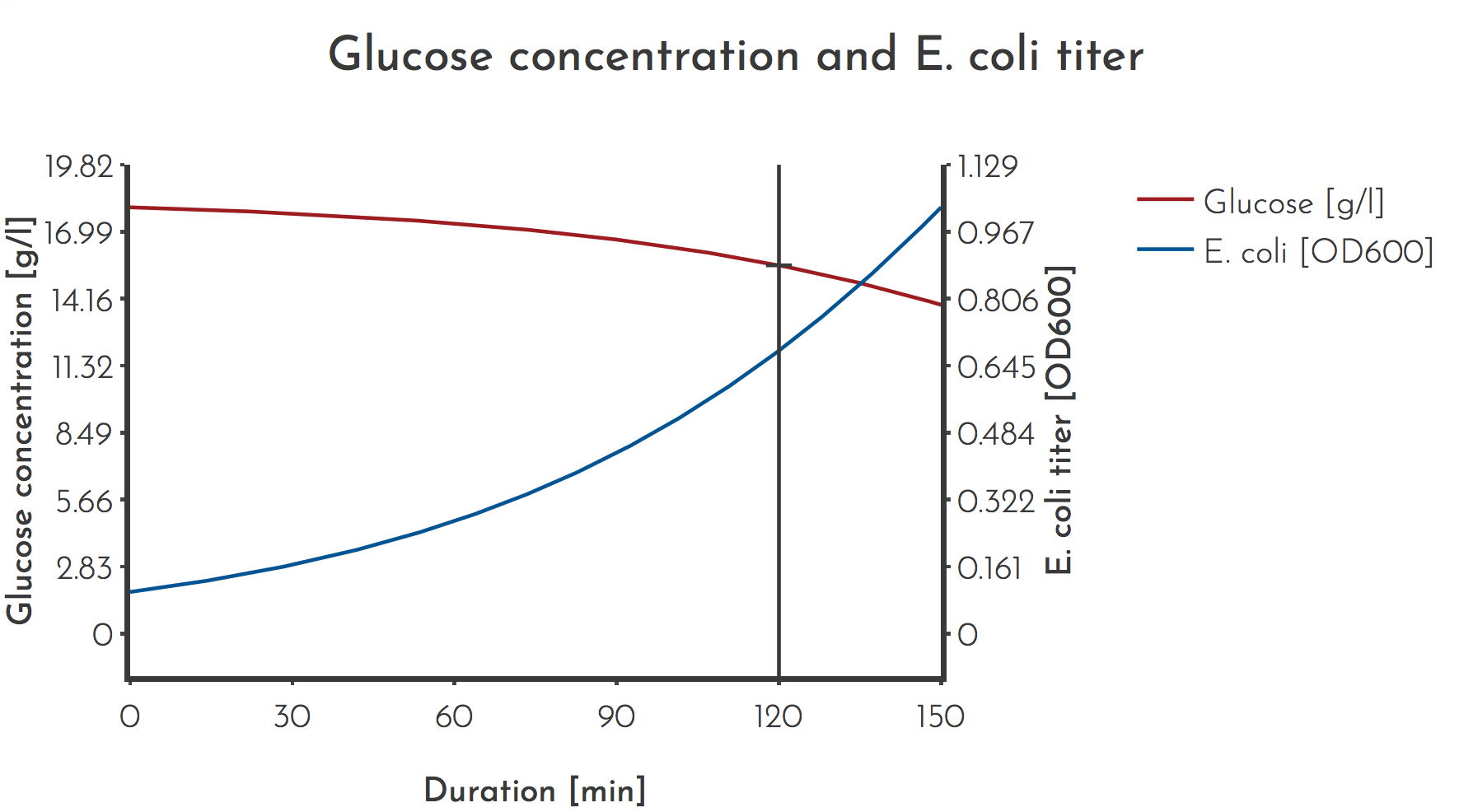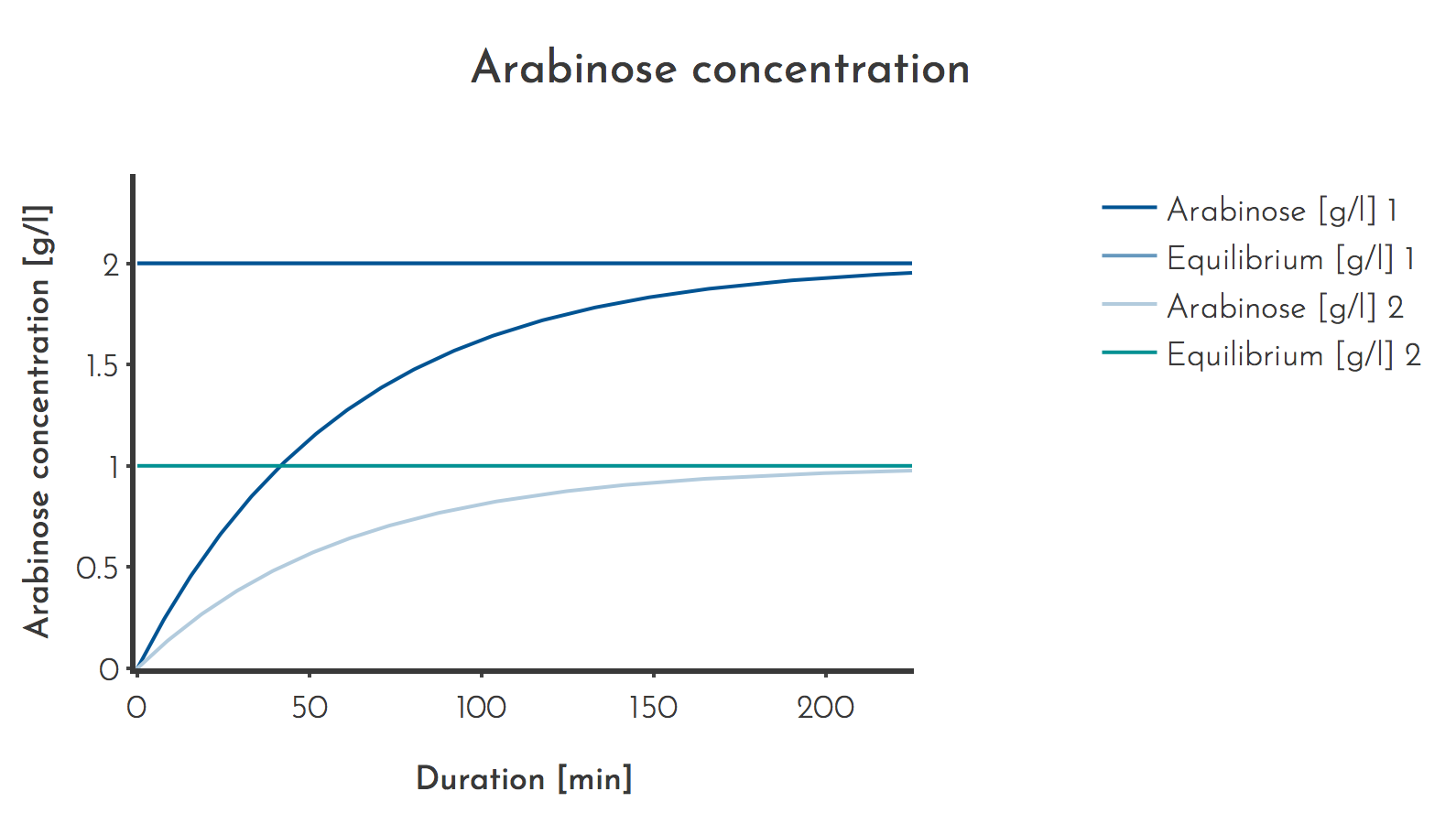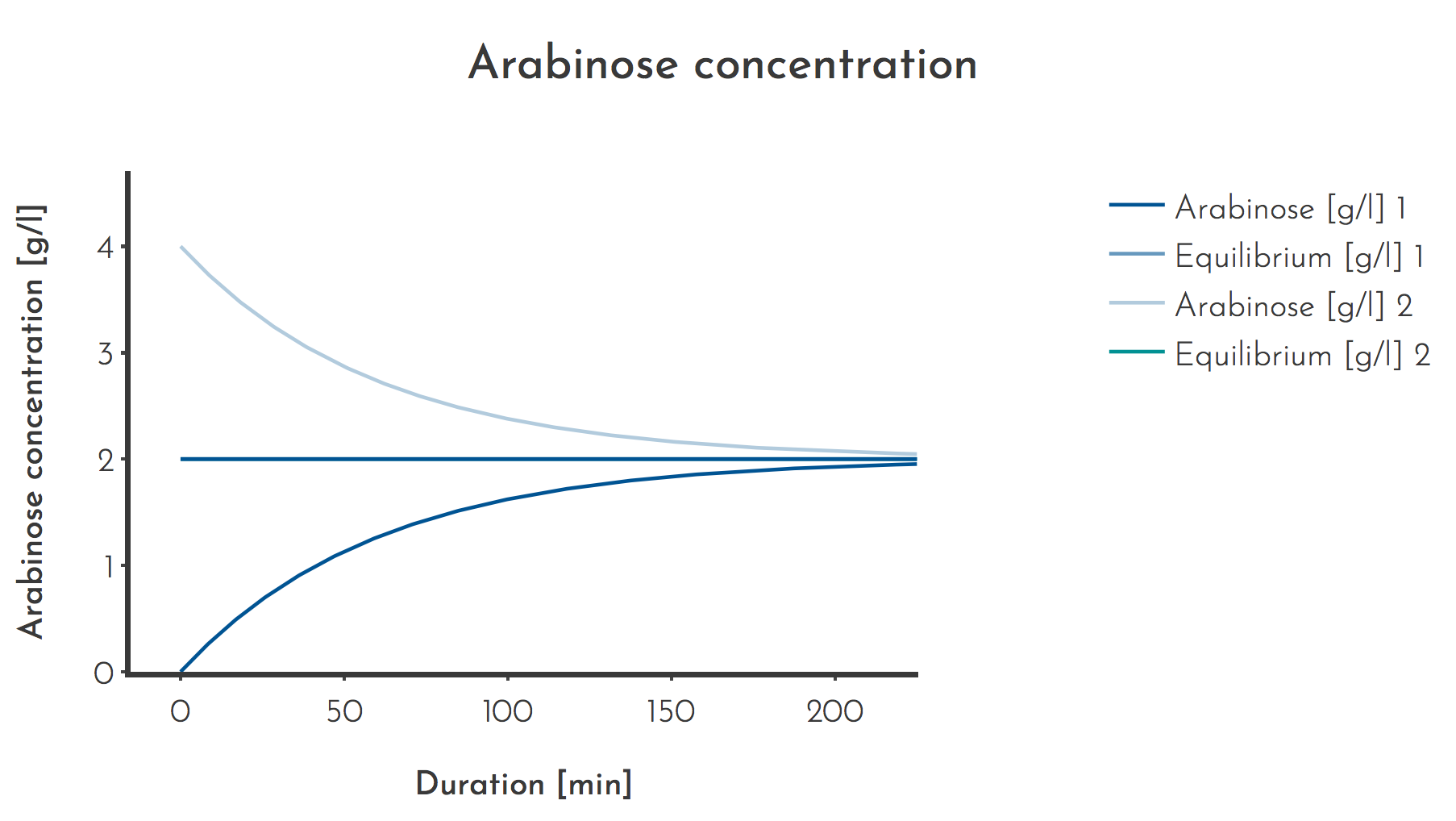# Team:Heidelberg/Model/Mutagenesis Induction

Modeling
Mutagenesis Induction
Mutagenesis plasmids are crucial to enable rapid mutation that makes continuous evolution possible in short time scales. The mutagenesis plasmids we used have a $P_{BAD}$ promotor that is arabinose inducible but suppressed by glucoseRN159. Consequently maintaining a high enough arabinose and low enough glucose concentration is important in order to have a strong induction of the mutagenesis plasmids necessary for a high mutation rate, which leads to a larger covered sequence space. The arabinose concentration is easier to tune since higher arabinose concentrations should simply induce the mutagenesis plasmids stronger while not being significantly metabolized. Glucose on the other hand as the primary carbon source is expected to be consumed by E. coli cells.
Therefore the glucose model uses ordinary differential equations (ODE) to model both the glucose and the E. coli concentration, assuming both are independend of each other. This is plausible because the medium used in the experiments contained carbon sources other than glucose. The glucose consumption rate per E. coli is assumed to be independent of the glucose concentration.

# Theory - Glucose

Modeling the glucose concentration in a PACE experiments lagoon is based on a set of steady state equations while the model for PREDCEL has to take the development over time into account. The glucose concentration in the turbidostat $c_{G_{T} }$ is increased with the incoming medium with a flow rate of $\Phi$ and a glucose concentration of $c_{G_{M} }$. It is decreased by with the medium that leaves the turbidostat with the same flow rate, but a glucose concentration of $c_{G_{T} }$. Additionally E. coli with a concentration of $c_{E}$ take up glucose at a rate of $q$.
$$\frac{\partial c_{G_{T} }(t)}{\partial t} = \Phi_{T} \cdot c_{G_{M} } - \Phi_{T} \cdot c_{G_{T} } - c_{E} \cdot q$$
In the case of a PACE experiment we can assume a dynamic equilibrium. That means that once a steady state is reached the concentrations stay the same and those concentrations are modeled here. First of all glucose is consumed in the turbidostat:
$$\frac{\partial c_{G_{T} }(t)}{\partial t} = 0$$
$$\Leftrightarrow c_{G_{T} } = c_{G_{M} } - \frac{c_{E} \cdot q}{\Phi_{T} }$$
$$\Leftrightarrow c_{G_{M} } (c_{G_{T} }) = c_{G_{T} } + \frac{c_{E} \cdot q}{\Phi_{T} }$$
When a lagoon with Volume $V_{L}$ and a flowrate of $\Phi_{L}$ is supplied by the turbidostat the glucose consumption in that lagoon can be modeled the same way. Because the E. coli titer, glucose concentration and flow rate into the lagoon are constant, a second steady state equilibrium can be assumed:
$$c_{G_{L} } = c_{G_{T} } - \frac{c_{E} \cdot q}{\Phi_{L} }$$
In the context of PACE mutagenesis plasmids are induced in the lagoons which stops growth of E. coli (see ), hence the E. coli titer is assumed to be the same as in the turbidostat. This simplifies the combination of both equations and results in the full equation
$$c_{G_{L} } = c_{G_{M} } - \frac{c_{E} \cdot q}{\Phi_{T} } - \frac{c_{E} \cdot q}{\Phi_{L} }$$
$$\Leftrightarrow c_{G_{M} } (c_{G_{L} }) = c_{G_{L} } + \frac{c_{E} \cdot q}{\Phi_{T} } + \frac{c_{E} \cdot q}{\Phi_{L} }$$
According to this the glucose concentration in the medium $c_{G_{M} }$has to be chosen so that it is the sum of the inteded glucose concentration in the lagoon $c_{G_{L} }$ and the amount of glucose that is consumed in both the turbidostat with flow rate $\Phi_{T}$ and the lagoon with flow rate $\Phi_{L}$.

When a PREDCEL experiment is carried out the steady state assumption does not apply as the glucose in a flask is consumed over time, resulting in end concentrations that differ from the initial ones.
The glucose concentration in a flask $c_{G_{F} }$ only changes by consumption since no glucose is added during PREDCEL. The consumption of glucose until a given timepoint equals to the integral over the E. coli concentration $c_{E}$ multiplicated with the glucose consumption factor $q$. That is the amount of glucose that is consumed by a given amount of E. coli during a given duration.
$$\frac{\partial c_{G_{F} }(t)}{\partial t} = q \cdot \int_{t_{0} }^{t} c_{E}(t) \: dt$$
At any point in time the current glucose concentration is simply the difference between the intial concentration and the glucose that was already consumed.
$$c_{G_{F} }(t) = c_{G_{F} }(t_{0}) - q \cdot \int_{t_{0} }^{t} c_{E}(t) \: dt$$
For the calculation of $c_{E}$ both exponential growth and logistic growth were assumed in two different models. Especially for high E. coli concentrations the logistic growth model should be more precise since it takes into account that there is an upper limit for the E. coli concentration.
Exponential growth is defined asmathmodelsib
$$\frac{\partial c_{E}(t)}{\partial t} = \frac{ln(2)}{t_{E} } \cdot c_{E}(t)$$
Introducing this term into the above equation gives
$$c_{G_{F} }(t)= c_{G_{F} }(t_{0}) -q \cdot \int_{t_{0} }^{t} c_{E}(t_{0}) \cdot exp\left(\frac{ln(2) \cdot t}{t_{E} }\right) dt$$
$$c_{G_{F} }(t)= c_{G_{F} }(t_{0}) -q \cdot \int_{t_{0} }^{t} c_{E}(t_{0}) \cdot exp\left(\frac{ln(2) \cdot t}{t_{E} }\right) dt$$
$$= c_{G_{F} }(t_{0}) - q \cdot c_{E}(t_{0}) \cdot t_{E} \cdot \left(exp\left(\frac{ln(2) \cdot t}{t_{E} }\right) - exp\left(\frac{ln(2) \cdot t_{0} }{t_{E} }\right)\right)$$
This results in the glucose starting concentration $c_{G_{F} }(t_{0})$ needed to reach a concentration of $c_{G_{f} }(t)$ afer a duration of $t$ being calculated by
$$c_{G_{F} }(t_{0}) = c_{G_{F} }(t) + q \cdot c_{E}(t_{0}) \cdot t_{E} \cdot \left(exp\left(\frac{ln(2) \cdot t}{t_{E} }\right) - exp\left(\frac{ln(2) \cdot t_{0} }{t_{E} }\right)\right)$$

Logistic growth is defined as
$$\frac{\partial c_{E}(t)}{\partial t} = \frac{ln(2)}{t_{E} } \cdot \frac{c_{c} - c_{E}(t)}{c_{E}(t)}$$
The analytical solution to this gives
$$c_{E}(t) = \frac{c_{c} }{1 + \big(\frac{c_{c} }{c_{E} (t_{0})} - 1\big) \cdot exp\big(-\frac{ln(2)}{t_{E} } \cdot t\big)}$$
If logistic growth is assumed, the term for $c_{E}(t)$ is substituted by expression above. Here $c_{c}$ is the capacity, the maximum concentration of E. coli under the present conditions.
$$c_{G_{F} } (t) = c_{G_{F} } (t_{0}) - q \int_{t_{0} }^{t} \frac{c_{c} }{1 + \big(\frac{c_{c} }{c_{E} (t_{0})} - 1\big) \cdot exp\big(-\frac{ln(2)}{t_{E} } \cdot t\big)} \: dt$$
$$= c_{G_{F} }(t_{0}) - \frac{q \cdot t_{E} \cdot c_{c} }{ln(2)} \cdot \Bigg(ln\bigg(c_{c} + c_{E}(t_{0})\cdot\Big(exp\big(\frac{ln(2)}{t_{E} } \cdot t \big) - 1\Big)\bigg) - ln\big(c_{c} \big)\Bigg)$$
This results in the glucose starting concentration $c_{G_{F} }(t_{0})$ needed to reach a concentration of $c_{G_{f} }(t)$ after a duration of $t$ being calculated by
$$c_{G_{F} }(t_{0}) = c_{G_{F} }(t) + \frac{q \cdot t_{E} \cdot c_{c} }{ln(2)} \cdot \Bigg(ln\bigg(c_{c} + c_{E}(t_{0})\cdot\Big(exp\big(\frac{ln(2)}{t_{E} } \cdot t \big) - 1\Big)\bigg) - ln\big(c_{c} \big)\Bigg)$$

Further calculations for simplification of entering data:
The glucose concentration in grams of dryweight is needed in order to work with the literature value of $q$. It can be calculated from the optical density at a wavelength of $\lambda=600 \: nm$ as
$$c_{E. coli_{DW} } = c_{E. coli_{OD600} } \cdot 0.36$$
according to Milo et al.Milo2009. The value of $q$ is
$$q = 0.183 \: g_{Glucose} \: g_{DW}^{-1} \: h^{-1} = 0.5083 \: g_{Glucose} \: OD600^{-1} \: h^{-1}$$
according to Neubauer et al.Neubauer2001. Because turbidostats are operated at a constant cell density, the flow rate $\Phi$ can be calculated from the generation time $t_{E}$ since the qualification of a turbidostat is that its dilution rate $\Phi_{T}$ equals the growth rate of the culture.
$$\Phi_{T} = \frac{ln(2)}{t_{E} }$$

Table 1: Variables and Parameters used for the calculation of the glucose and E. coli concentrations. List of all paramters and variables used in the analytical solution of this model and in the interactive webtool that is provided.

Symbol Value and Unit Explanation
$c_{G_{T} }$ [g/ml] or [mmol/ml] Glucose concentration in Turbidostat
$c_{G_{M} }$ [g/ml] or [mmol/ml] Glucose concentration in medium
$c_{G_{L} }$ [g/ml] or [mmol/ml] Glucose concentration in lagoon
$t$ [min] Time
$\Phi_{T}$ [ml/min] Flow rate through Turbidostat
$\Phi_{L}$ [ml/min] Flow rate through Lagoon
$c_{E}$ [cfu/ml] or OD600 E. coli concentration
$q$ $[g_{glucose} \: g_{DW}^{-1} h^{-1}]$ Glucose consumption by E. coliNeubauer2001
$t_{E}$ [min] E. coli generation time

# Practice - Glucose

Many values can be taken from literature but since phage infection has an effect on the performance of E. coli, the maximum capacity for the E. coli concentration in our setup had to be determined with our conditions. For one accessory plasmid the optical density was determined with and without phage infection over more then ten hours. Both cultures reached the maximum density after 510 min with an OD600 of 6.09 for the phage free culture and 5.133 for the infected culture. In the context of PREDCEL these values may serve as an estimation of the maximum E. coli capacity.Figure 1: E. coli titer measurement for estimation of the E. coli capacity
The experiment was carried out in triplicates, the mean is plotted. If the optical density was higher than 1, samples were diluted and measured. The accessory plasmid (AP_dark) carries a phage shock promotor under which gene III of the phage is expressed upon infection. The culture volume was 20 ml.Figure 2: Modeled Glucose and E. coli titer for a representative PREDCEL experiment
Most PREDCEL experiments were carried out with a glucose concentration of $c_{G_{M} } = 100 \: mmol/l$, an initial OD of $c_{E} = 0.1$, usually phage was added at $t = 120 \: min$.
Calculated with the Interactive Webtool of this model

# Theory - Arabinose

Arabinose functions as an inducer for the mutagenesis plasmids and it is assumed to not be degraded by E. coli in this model. Thus in a PREDCEL experiment arabinose concentration $c_{A}$ is constant over time, because neither the total volume changes nor the amount of arabinose in the flask. $$\frac{\partial c_{A_{L} } }{\partial t} = 0$$ and the concentration is the starting concentration: $$c_{A_{L} }(t) = c_{A_{L} }(t_{0})$$ If the arabinose concentration is modeled for a lagoon supplied by a turbidostat with a separate supply of arabinose solution, the system converges to a steady state, when turbidostat volume $V_{T}$, arabinose influx $\Phi_{S}$ and flow rate of turbidostat $\Phi_{T}$ are constant. In that case $$\frac{\partial c_{A} }{\partial t} = 0$$ is true. The change in lagoon arabinose concentration $c_{A_{L} }$ is described by $$\frac{\partial c_{A_{L} }(t)}{\partial t} = \Phi_{S} \cdot c_{A_{S} } - \Phi_{L} \cdot c_{A_{L}(t)}$$ $\Phi_{S}$ and $\Phi_{L}$ are measured relative to the lagoon volume. The arabinose concentration in the lagoon $c_{A_{L} }$ can then be calculated using the concentration of the arabinose solution with which the lagoon is supplied $c_{A_{S} }$. $$c_{A_{L} } = \frac{\Phi_{S} }{\Phi_{L} } \cdot c_{A_{S} }$$ However, in some cases it may be relevant to estimate when a given percentage of the equilibrium concentration is reached. To make statements about that, the differential equation is solved to $$c_{A_{L} }(t) = \frac{\Phi_{S} }{\Phi_{L} } \cdot c_{A_{S} } - \big(\frac{\Phi_{S} }{\Phi_{L} } \cdot c_{A_{S} } - c_{A_{L} }(t_{0})\big) \cdot e^{-\Phi_{L} t}$$

Table 2: Variables and Parameters used for the calculation of the glucose and E. coli concentrations List of all paramters and variables used in the numeric solution of this model. Also have a look at the interactive webtool based on this model

Symbol Value and Unit Explanation
$c_{A_{L} }$ [g/ml] Arabinose concentration in Lagoon
$c_{A_{L}(t_{0}) }$ [g/ml] Initial Arabinose concentration in Lagoon
$\Phi_{S}$ [lagoon volumes/h] Flow rate of inducer supply relative to lagoon volume
$\Phi_{L}$ [lagoon volumes/h] Flow rate of lagoon

# Practice - Arabinose

In all performed PACE experiments the inducer flow rate was $\Phi_{S} = 2 ml/h$ and the lagoon volume was $V_{L} = 100 ml$, resulting in a normalised $\Phi_{S} = 0.02$.
In the first experiments, $c_{A_{S} }$ was set to 50 g/l, corresponding to 333 mmol/l. The conditions result in $c_{A_{L} } = 1 g/l$, or 6 mmol/l.
Later the inducer concentration was doubled to $c_{A_{S} } = 100 g/l$, resulting in a lagoon concentration of $c_{A_{L} } = 2 g/l$ or 12 mmol/l.
To circumvent low arabinose concentrations in the beginning, the experiment can be started with starting concentrations above the equilibrium concentration. Since no negative effects of high arabinose concentrations on the outcome of PACEFigure 3: Arabinose concentrations used for PACE
Initially a lower inducer concentration was used (Plot 2), later it was doubled to 100 g/l. The prediction shows that it takes 3 h until 90 % of the equilibrium concentration is reached.
Calculated with the Interactive Webtool of this modelFigure 4: Effect of starting concentrations higher than the equilibrium concentrations
The Arabinose concentration approaches the equilibrium concentration from either above (Plot 1) or below (Plot 2). Assumed values are $c_{A_{S} } = 100 \: g/l$, $\Phi_{L} = 1$ lagoon volumes/h, $\Phi_{A} = 0.02$ lagoon volumes/h, $t = 120 min$, $c_{A_{L} } (t_{0}) = 0 g/l$ or $c_{A_{L} }(t_{0}) 4 g/l$
Calculated with the Interactive Webtool of this model